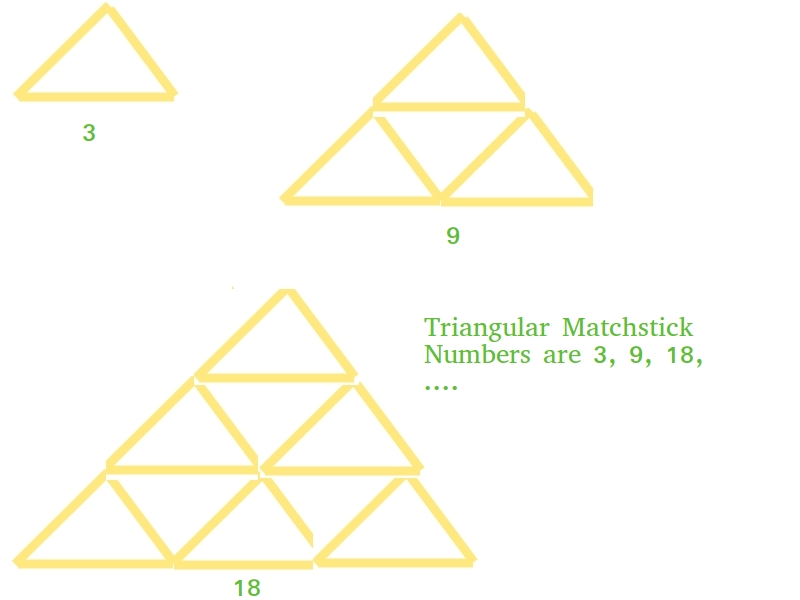Related Articles

# Python Program for Triangular Matchstick Number

• Last Updated : 03 Dec, 2018

Given a number X which represents the floor of a matchstick pyramid, write a program to print the total number of matchstick required to form a pyramid of matchsticks of x floors.

Examples:

```Input : X = 1
Output : 3
Input : X = 2
Output : 9
```

This is mainly an extension of triangular numbers. For a number X, the matchstick required will be three times of X-th triangular numbers, i.e., (3*X*(X+1))/2`# Python program to find X-th triangular``# matchstick number`` ` `def` `numberOfSticks(x):``    ``return` `(``3` `*` `x ``*` `(x ``+` `1``)) ``/` `2``     ` `# main()``print``(``int``(numberOfSticks(``7``)))`
Output:
```84
```

Please refer complete article on Triangular Matchstick Number for more details!

My Personal Notes arrow_drop_up